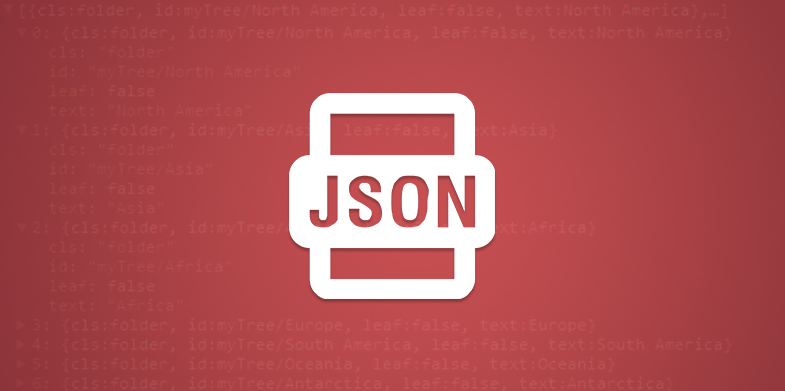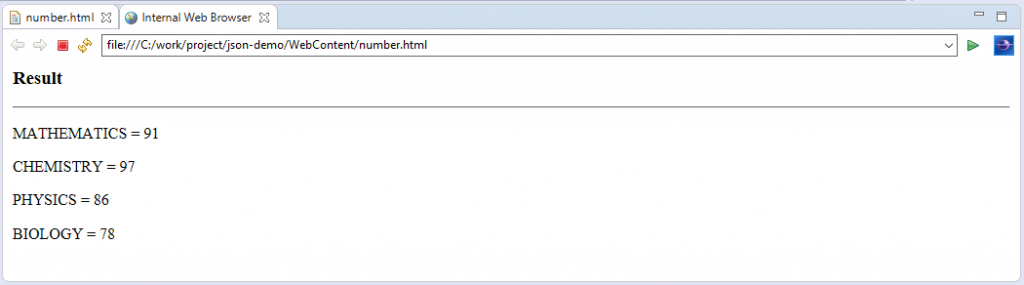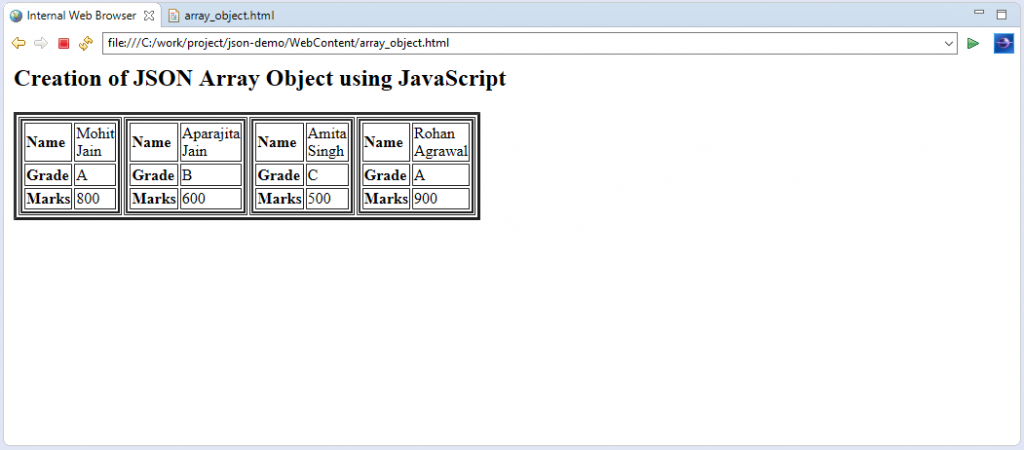# Learn about the Creation of Various JSON Objects

0
3569In the last chapter, we discussed about the various data types permitted in JSON format along with its overview and example. In this chapter, we are going to discuss about the JSON Objects in detail. We can create JSON object with JavaScript. The following are the ways to create JSON objects with the help of JavaScript.

Creation of JSON Objects
The following are the different types of JSON Objects which are frequently used in the JSON format documents.

1. Empty JSON Object: An empty object, which can be created with the syntax as “var JSON_Object = {};”
2. New JSON Object: A new Object which can be created with the syntax as “var JSON_Object = new Object ();”
3. Simple JSON Object: A simple object with attributes as subject name and marks of data type as string and number respectively. The attribute values are accessed by using ‘.’ Operator as shown below.

```<html>
<title>JSON example</title>
<script language="javascript">
var subject_marks = {maths: 91, chemistry: 97, physics: 86, biology: 78};
document.write (" <h3> Result </h3> ");
document.write (" <hr> ");
document.write (" <p> MATHEMATICS = " + subject_marks.maths + "</p>");
document.write (" <p> CHEMISTRY = " + subject_marks.chemistry + "</p>");
document.write (" <p> PHYSICS = " + subject_marks.physics + "</p>");
document.write (" <p> BIOLOGY = " + subject_marks.biology + "</p>");
</script>
<body>
</body>
</html>```

Output
When we execute above HTML code on browser, then we will observe the following output.4. Array JSON Objects
• As explained in the last chapter, a JSON array object has the following properties.
• An array is an ordered collection of values.
• An array is represented as an enclosure in square brackets i.e. begins with. [. and ends with.]…
• The values in an array are separated by, (comma).
• Indexing in an array can be started at 0 or 1.
• Arrays are recommended to be used when the key names are sequential integers.
In the following example, we are going to shows the creation of two array objects with the help of JavaScript using JSON format.

```<html>
<title>Array object creation in JSON using JavaScript </title>
<script language = "javascript" >
document.writeln("<h2>Creation of JSON Array Object using JavaScript</h2>");

var student = { "BE" : [
{ "Name"  : "Mohit Jain", "grade" : "A", "marks" : 800 },
{ "Name"  : "Aparajita Jain", "grade" : "B", "marks" : 600  }],

"TE"  : [
{ "Name"  : "Amita Singh", "grade" : "C", "marks" : 500  },
{ "Name"  : "Rohan Agrawal", "grade" : "A", "marks" : 900  }]
}

var i = 0
document.writeln("<table border = '3'><tr>");

for(i = 0; i<student.BE.length; i++){
document.writeln (" <td> ");
document.writeln (" <table border = '2' width = 100 > ");
document.writeln (" <tr> <td> <b> Name </b> </td> <td width = 40>" + student.BE[i].Name + " </td> </tr> ");
document.writeln (" <tr> <td> <b> Grade </b> </td> <td width = 30>" + student.BE[i].grade + " </td> </tr> " );
document.writeln (" <tr> <td> <b> Marks </b> </td> <td width = 30>" + student.BE[i].marks + " </td> </tr> ");
document.writeln (" </table> ");
document.writeln (" </td> ");
}

for(i = 0; I < student.TE.length; i++){
document.writeln(" <td> ");
document.writeln(" <table border = '2' width = 100 > ");
document.writeln(" <tr> <td> <b> Name </b> </td> <td width = 40>" + student.TE[i].Name + " </td> </tr> ");
document.writeln(" <tr> <td> <b> Grade </b> </td> <td width = 30>" + student.TE[i].grade+" </td> </tr> ");
document.writeln (" <tr> <td> <b> Marks </b> </td> <td width = 30>" + student.TE[i].marks + " </td> </tr> ");
document.writeln (" </table> ");
document.writeln (" </td> ");
}

document.writeln (" </tr> </table> ");

</script>